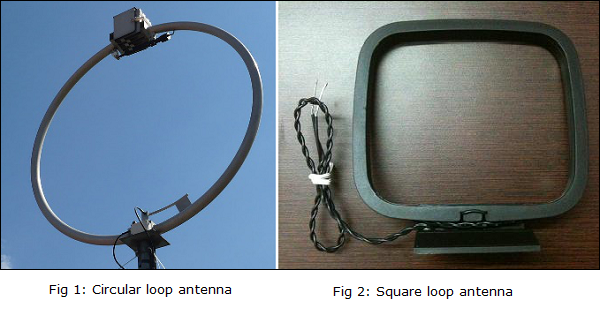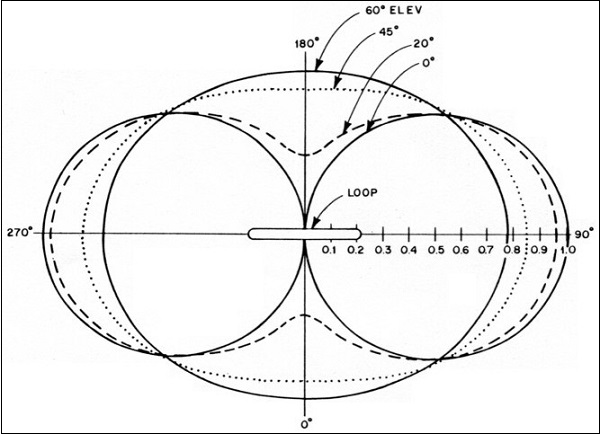# Antenna Theory - Loop

An RF current carrying coil is given a single turn into a loop, can be used as an antenna called as loop antenna. The currents through this loop antenna will be in phase. The magnetic field will be perpendicular to the whole loop carrying the current.

### Frequency Range

The frequency range of operation of loop antenna is around 300MHz to 3GHz. This antenna works in UHF range.

## Construction & Working of Loop Antennas

A loop antenna is a coil carrying radio frequency current. It may be in any shape such as circular, rectangular, triangular, square or hexagonal according to the designer’s convenience.

Loop antennas are of two types.

• Large loop antennas
• Small loop antennas

### Large loop antennas

Large loop antennas are also called as resonant antennas. They have high radiation efficiency. These antennas have length nearly equal to the intended wavelength.

$$L =\lambda$$

Where,

• L is the length of the antenna

• λ is the wavelength

The main parameter of this antenna is its perimeter length, which is about a wavelength and should be an enclosed loop. It is not a good idea to meander the loop so as to reduce the size, as that increases capacitive effects and results in low efficiency.

### Small loop antennas

Small loop antennas are also called as magnetic loop antennas. These are less resonant. These are mostly used as receivers.

These antennas are of the size of one-tenth of the wavelength.

$$L =\frac{\lambda}{10}$$

Where,

• L is the length of the antenna

• λ is the wavelength

The features of small loop antennas are −

• A small loop antenna has low radiation resistance. If multi-turn ferrite core constructions are used, then high radiation resistance can be achieved.

• It has low radiation efficiency due to high losses.

• Its construction is simple with small size and weight.

Due to its high reactance, its impedance is difficult to match with the transmitter. If loop antenna have to act as transmitting antenna, then this impedance mis-match would definitely be a problem. Hence, these loop antennas are better operated as receiver antennas.

## Frequently Used Loops

Small loop antennas are mainly of two types −

• Circular loop antennas
• Square loop antennas

These two types of loop antennas are mostly widely used. Other types (rectangular, delta, elliptical etc.) are also made according to the designer specifications.The above images show circular and square loop antennas. These types of antennas are mostly used as AM receivers because of high Signal-to-noise ratio. They are also easily tunable at the Q-tank circuit in radio receivers.

## Polarization of Loop

The polarization of the loop antenna will be vertically or horizontally polarized depending upon the feed position. The vertical polarization is given at the center of the vertical side while the horizontal polarization is given at the center of the horizontal side, depending upon the shape of the loop antenna.

The small loop antenna is generally a linearly polarized one. When such a small loop antenna is mounted on top of a portable receiver, whose output is connected to a meter, it becomes a great direction finder.

The radiation pattern of these antennas will be same as that of short horizontal dipole antenna.The radiation pattern for small, high-efficiency loop antennas is shown in the figure given above. The radiation patterns for different angles of looping are also illustrated clearly in the figure. The tangent line at 0° indicates vertical polarization, whereas the line with 90° indicates horizontal polarization.

The following are the advantages of Loop antenna −

• Compact in size
• High directivity

The following are the disadvantages of Loop antenna −

• Impedance matching may not be always good
• Has very high resonance quality factor

### Applications

The following are the applications of Loop antenna −

• Used in RFID devices
• Used in MF, HF and Short wave receivers
• Used in Aircraft receivers for direction finding
• Used in UHF transmitters